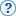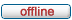# forum.alglib.net

ALGLIB forum
 It is currently Fri Jun 09, 2023 7:01 pm

 All times are UTC

### Forum rules

1. This forum can be used for discussion of both ALGLIB-related and general numerical analysis questions
2. This forum is English-only - postings in other languages will be removed.

 Print view Previous topic | Next topic
Author MessagePost subject: Help on Alglib lsfit package used to optimize monte-carloPosted: Fri Dec 16, 2016 3:36 pmJoined: Fri Dec 16, 2016 3:21 pm
Posts: 1
Hi all,

I'm trying to develop a model using monte-carlo to generate a set of simulated data. These simulated data is then compared to the experimental data to extract the optimum parameters, which totals 9. This is what I have tried.

Code:
void function_cx_1_func(const alglib::real_1d_array &c, const alglib::real_1d_array &x, double &func, void *ptr)
{
//  The arguments follow the format of the alglib's lsfit subpackage
//  This function requires the func to return a double.
//  c is a 1d array of parameters
//  x is temperature, which is the only variable in this case
//  ptr is NULL in by the package's default.
//  cout << "iterred" << endl;
map <double,double> simulation_result;
if (
//(gmm != c) ||
(Tm != c) ||
(g31 != c) ||
(g32 != c) ||
(gg31 != c) ||
(gg32 != c) ||
(bn != c) ||
(bu != c) ||
(mn != c) ||
(mu != c)
)
{
//gmm = c;
Tm = c;
g31 = c;
g32 = c;
gg31 = c;
gg32 = c;
bn = c;
bu = c;
mn = c;
mu = c;
Tjumpave = Tjump_ave();
}
double T = 5;
//    for (mat::iterator entry = Tjumpave.begin(); entry != Tjumpave.end(); ++entry) {
//        simulation_result[T] = *entry;
//        T += 5;
//    }
for (int i = 0; i != 9; ++i)
{
simulation_result[T] = Tjumpave(0,i);
T += 5;
}
func = simulation_result[x];
}
vector<double> params_optim()
{
//  Optimization using lsfit
//  The params_optim function optimizes the values of the simulation parameters
//  The parameters are: Tm, g31, g32, gg31, gg32, bn, bu, mn, mu (9)
vector<double> par = {1,1,1,1,1,1,1,1,1};
try
{
alglib::real_2d_array T = "[,,,,,,,,,,,,,,,,,,]";  //  Temperatures in experiments (independent var)
alglib::real_1d_array exp = "[346.301,346.312,346.432,346.394,346.471,346.605,346.797,346.948,347.121,347.384,347.626,348.08,348.561,349.333,350.404,351.761,352.975,354.17,354.809]";  //  Exp data
alglib::real_1d_array params = "[80,550,400,0,0,347,354,0.013,0.012]"; //  in the order given above
alglib::real_1d_array bndl = "[75,-INF,-INF,-INF,-INF,345,350,0.005,0.005]";  //  lower bounds of params
alglib::real_1d_array bndu = "[83,+INF,+INF,+INF,+INF,349,360,0.030,0.030]";  //  upper bounds of params
//  Define the stopping criteria
double epsf = 0.0; //  stops when the parameter value of the next iter is less than epsf away from the previous's
double epsx = 0.000001; //  stops when the scaled step vector (scaled by LSfitsetscale) is <= epsx
alglib::real_1d_array s = "[1e+1,1e+2,1e+2,1e+2,1e+2,1e+2,1e+2,1e-2,1e-2]"; //  set the scales (orders of magnitude) of the parameter
alglib::ae_int_t info; //  to store how the "more_information code number"
alglib::ae_int_t maxits = 100; //  set the number of iteration to perform
alglib::lsfitstate state; //  store the "state"
alglib::lsfitreport rep;  //  store the report
double diffstep = 5; //  step to take for each param: too high, miss points. too low, higher accuracy but more round-off error

alglib::lsfitsetscale(state, s);
alglib::ae_int_t n = 19;  //  number of points
alglib::ae_int_t m = 1;  //  number of dimension (only temperature)
alglib::ae_int_t k = 9;  //  number of parameters to fit (10)
alglib::lsfitcreatef(T, exp, params, n,m,k, diffstep, state); //  create a fitting instance
alglib::lsfitsetbc(state, bndl, bndu);
alglib::lsfitsetcond(state, epsf, epsx, maxits); //  before fitting, set a stopping point
alglib::lsfitfit(state, function_cx_1_func); //  fit
alglib::lsfitresults(state, info, params, rep);
par = { params,params,params,params,params,params,params,params,params };
}
catch (const alglib::ap_error &e)
{
printf("error msg: %s\n", e.msg.c_str());
}
return par;
}

More information about the monte-carlo. This function is defined within the Tjumpave() and returns an Armadillo matrix of simulated data for every set of parameters. Then because func requires double, I mapped each simulated data point to my independent var (temperature) and set func = data at that temperature.

Overall, the code didn't fail to run, but the results is just the same as the initial solutions. And I know the initial solution is ball park but not exactly the same as the initial value, so I'm not sure if I had the alglib function correct. Please help me in the right direction.

TopDisplay posts from previous: All posts1 day7 days2 weeks1 month3 months6 months1 year Sort by AuthorPost timeSubject AscendingDescending

 All times are UTC

#### Who is online

Users browsing this forum: No registered users and 20 guests

 You cannot post new topics in this forumYou cannot reply to topics in this forumYou cannot edit your posts in this forumYou cannot delete your posts in this forumYou cannot post attachments in this forum

Search for: